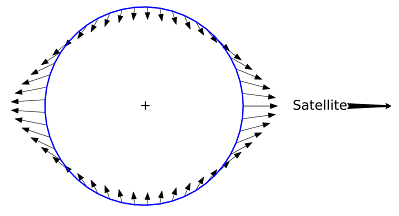### Roche Limit: The Radius of Disintegration

_____________________________________

I think most guys can recall memorable father-son activities they did growing up - for me it was primarily golf, but another one I distinctly remember is watching old-school Chinese kung fu movies with my dad.  Most of them were exactly as you'd expect: awful, complete with ketchup blood, goofy one-liners, dreadful acting. Of course it was fun nonetheless, and some of them were halfway decent! (check out Snake in the Eagle's Shadow and Drunken Master: two classics by a young Jackie Chan)

But to this day, I still don't really like watching Chinese-made action movies: the modern ones are chock-full of heavy-handed CGI, not-so-subtle propaganda, and more bad acting (Wolf Warriors is a perfect example of a film that was crazy popular in China, but that I found abysmal). So when a good friend of mine recommended I watch The Wandering Planet, I was pretty skeptical and only agreed after I learned the movie is based off a short story by Cixin Liu, whose Three Body Problem trilogy is one of the best sci-fi stories I've ever read. The movie was pretty mediocre, but it did introduce me to a neat concept in orbital mechanics: the Roche limit!哎呀 - AIYAAAAAAAAAA!!!!!!Ladies and gentlemen, I present to you the greatest death scene in the history of cinema

The basic premise of The Wandering Planet is that the sun is dying, so humanity builds enormous planet-moving thrusters to eject Earth from the solar system and find a new host star. But a malfunction en route causes Earth to be captured by Jupiter's gravity, leading to a desperate effort to restart the engines before Earth falls within Jupiter's Roche limit and gets ripped apart by tidal forces**. The Roche limit is a real phenomenon; calculated in 1848 by French mathematician Edouard Roche, it's the distance from a central body within which a satellite would disintegrate as tidal forces overcome the satellite's gravitational self-attraction. Said differently, matter inside the Roche limit cannot coalesce into moons, but will instead break apart and possibly form rings. Now, the Roche limit is different for every central body-satellite combination. The question is, what factors does it depend on?

Tidal force**: the gravitational effect that stretches a satellite in the direction of the central body, because the parts of the satellite closer to the central body are more strongly attracted than the parts that are farther away. If this disparity is greater than the gravitational self-attraction that holds the satellite together, it can rip the satellite apartDiagram of tidal forces, showing the satellite being stretched in the direction of the central bodyVisualizing the Roche limit

The answer is that it mostly comes down to two factors: the ratio between the densities of the central body and the satellite, and the rigidity of the satellite. For the densities, although determining these values is challenging in practice (we've got pretty good values for the densities of the Earth and the moon, but what about some random unexplored body like Neptune's moon Thalassa?), mathematically it's a simple input. It's the rigidity of the satellite that makes determining the Roche limit extremely difficult.

Consider the simplest possible scenario: a perfectly rigid spherical satellite (meaning it will maintain its shape exactly, right up until the moment it breaks apart). In this case, the formula for calculating the Roche limit is given as:

$d = R_M (2 \frac{\rho_M}{\rho_m})^\frac{1}{3}$

Whereby

$d$: Roche limit    $R_M$: radius of the central body    $\rho_M$: density of the central body    $\rho_m$: density of the satellite

Now consider the opposite extreme: a perfectly fluid spherical satellite (imagine an enormous spherical water droplet orbiting a planet). In this case, the formula for calculating the Roche limit is given as:

$d \approx 2.44 R_M (\frac{\rho_M}{\rho_m})^\frac{1}{3}$

You'll notice the approximate sign for the fluid formula: even in this already heavily simplified example, the Roche limit can't be determined by an exact algebraic formula (see the Wikipedia page for an in-depth derivation of the Roche limit formulas)Great animation from BBC's The Planets showing an icy moon disintegrating as it passes the Roche limit

So if these two formulas can only describe our highly-contrived scenarios (perfectly rigid / perfectly fluid) and ignore other factors like the satellite's rotation or tensile strength, what good are they? Well, since actual satellites would fall somewhere in between being perfectly rigid and perfectly fluid, we can use them to estimate what the upper and lower bounds of the Roche limit would be! Taking the familiar example of the Earth and the moon, knowing that Earth's radius is 6,378km, Earth's mean density is 5,513kg/m^3, and the moon's mean density is 3,346kg/m^3, we can determine that the Earth-moon Roche limit is 9,492km based on the rigid formula and 18,381km based on the fluid formula, and that the actual Roche limit likely lies somewhere in between. Given that the moon orbits at about 384,399km (21x further than the more conservative fluid Roche limit formula), we can feel pretty confident that the moon isn't going to disintegrate anytime soon!Other selected examples of Roche limits for various central bodies-satellites

To see more detailed and accurate Roche limit computations, definitely check out the Wikipedia!